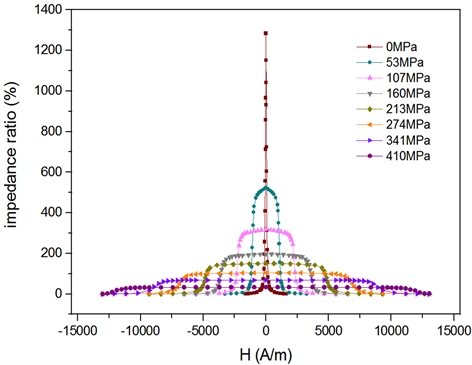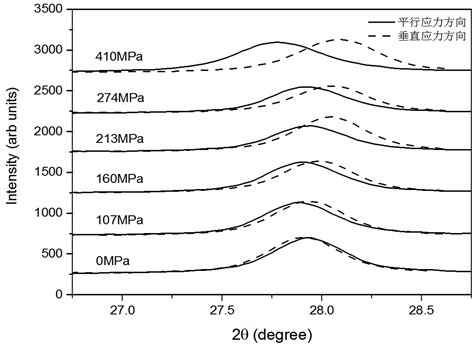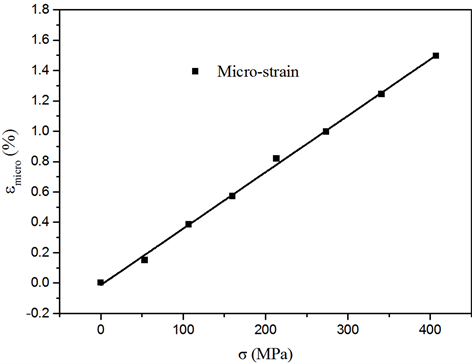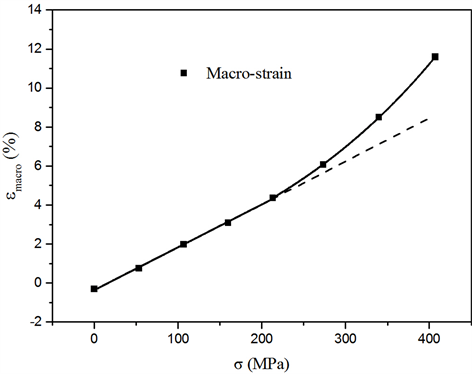# Fe基合金薄带应力感生磁各向异性非线性关系研究Nonlinear Relationship of Stress-Induced Magnetic Anisotropy in Fe-Based Alloy Ribbons

DOI: 10.12677/MS.2021.112015, PDF, HTML, XML, 下载: 62  浏览: 112  国家自然科学基金支持

Abstract: The magnetic anisotropy, lattice anisotropy and macroscopic changes of Fe-Cu-Nb-Si-B amorphous alloy samples under free and tensile stress annealing conditions were studied by in-situ synchro-tron X-ray diffractometer, HP4294A impedance analyzer and elongation in-situ observation tools. The results show that the lattice anisotropy of the sample has a linear relationship with the applied tensile stress, and the magnetic anisotropy has an exponential relationship with the applied tensile stress. The magnetic anisotropy of the sample annealed with tensile stress is caused by the change of the crystal lattice anisotropy of the sample and the exchange coupling between neighboring nanocrystalline grains.

1. 引言

2. 实验

$\frac{\Delta Z}{Z}=\frac{{Z}_{{H}_{ex}}-{Z}_{{H}_{\mathrm{max}}}}{{Z}_{{H}_{\mathrm{max}}}}×100%$ (1)

${H}_{k}=\frac{{H}^{+}-{H}^{-}}{2}$ (2)

3. 结果与讨论

3.1. 不同退火样品的巨磁阻抗与磁各向异性Figure 1. GMI curves of samples annealed at 540˚C under different stresses

${\left(\frac{\Delta Z}{Z}\right)}_{\mathrm{max}}=694.58{\text{e}}^{-\frac{\sigma }{28.77}}+586.85{\text{e}}^{-\frac{\sigma }{152.77}}+2.48$ (3)

${H}_{k}=7863.34{\text{e}}^{\frac{\sigma }{444.27}}-7781.57$ (4)Figure 2. The relationship between the maximum giant magneto-impedance ratio, magnetic anisotropy field and stress of the sample

3.2. 不同应力退火样品的微观结构

3.3. 样品的宏观应变与微观应变

${\epsilon }_{micro}=\frac{{d}_{//}-{d}_{\perp }}{{d}^{0MPa}}$ (5)Figure 3. (200) peak position changes of free annealing and stress annealing samples in different directions

${\epsilon }_{micro}=0.0037\sigma -0.0131$ (6)

${\epsilon }_{macro}=\frac{\Delta l}{{l}_{0}}$ (7)

${\epsilon }_{macro}=0.02\sigma -0.36$ (8)

${\epsilon }_{macro}=5.87{\text{e}}^{\frac{\sigma }{371.93}}-6.03$ (9)Figure 4. The relationship curve between micro strain and stress of the sampleFigure 5. The relationship curve between macro strain and stress of the sample

$D=\frac{k\lambda }{B\mathrm{cos}\theta }$ (10)

4. 结论

540℃退火的样品感生的磁各向异性与外加应力呈指数变化，样品的微观应变与外加应力满足线性关系。外加应力进行540℃退火时，非晶基底的蠕变会造成纳米晶横向之间距离减小，近邻纳米晶粒间的交换耦合作用增大，纵向纳米晶间隙增大，近邻纳米晶粒间的交换耦合作用减小，从而产生纳米晶分布各向异性，所以应力感生的磁各向异性中84%来自于外加应力退火感生的纳米晶晶格各向异性，其余16%是来自于无法被回火所消除的纳米晶分布各向异性。

  Yoshizawa, Y., Oguma, S. and Yamauchi, K. (1988) New Fe-Based Soft Magnetic Alloys Composed of Ultrafine Grain Structure. Journal of Applied Physics, 64, 6044-6046. https://doi.org/10.1063/1.342149  Herzer, G. (1989) Grain Structure and Magnetism of Nanocrystalline Ferromagnets. IEEE Transactions on Magnetics, 25, 3327-3329. https://doi.org/10.1109/20.42292  Herzer, G. (1990) Grain Size Dependence of Coercivity and Permeability in Nanocrystalline Ferromagnets. IEEE Transactions on Magnetics, 26, 1397-1402. https://doi.org/10.1109/20.104389  Allia, P., Baricco, M., Tiberto, P. and Vinai, F. (1993) Kinetics of the Amorphous-to-Nanocrystalline Transformation in Fe73.5Cu1Nb3Si13.5B9. Journal of Applied Physics, 74, 3137-3143. https://doi.org/10.1063/1.354581  Kraus, L., Závěta, K., Heczko, O., et al. (1992) Magnetic Anisotropy in As-Quenched and Stress-Annealed Amorphous and Nanocrystalline Fe73.5Cu1Nb3Si13.5B9 Alloys. Journal of Mag-netism & Magnetic Materials, 112, 275-277. https://doi.org/10.1016/0304-8853(92)91172-P  Fukunaga, H., Furukawa, N., Tanaka, H., et al. (2000) Nanostructured Soft Magnetic Material with Low Loss and Low Permeability. Journal of Applied Physics, 87, 7103-7105. https://doi.org/10.1063/1.372944  Herzer, G. (1992) Nanocrystalline Soft Magnetic Materials. Journal of Magnetism and Magnetic Materials, 112, 258-262. https://doi.org/10.1007/978-94-011-2054-8_12  Yoshizawa, Y. and Yamauchi, K. (1989) Effects of Mag-netic Field Annealing on Magnetic Properties in Ultrafine Crystalline Fe-Cu-Nb-Si-B Alloys. IEEE Transactions on Magnetics, 25, 3324-3326. https://doi.org/10.1109/20.42291  Herzer, G. (1994) Creep Induced Magnetic Anisotropy in Nanocrystalline Fe-Cu-Nb-Si-B Alloys. IEEE Transactions on Magnetics, 30, 4800-4802. https://doi.org/10.1109/20.334226  Neuweiler, A., Hofmann, B. and Kronmüller, H. (1996) Approach to Magnetic Saturation in Nanocrystalline and Amorphous Fe73.5Cu1Nb3Si13.5B9. Journal of Magnetism & Magnetic Materials, 153, 28-34. https://doi.org/10.1016/0304-8853(95)00533-1  Ohnuma, M., Hono, K., Yanai, T., et al. (2005) Origin of the Magnetic Anisotropy Induced by Stress Annealing in Fe-Based Nanocrystalline Alloy. Applied Physics Letters, 86, Article ID: 152513. https://doi.org/10.1063/1.1901807  Ohnuma, M., Hono, K., Yanai, T., et al. (2003) Direct Evidence for Structural Origin of Stress-Induced Magnetic Anisotropy in Fe-Si-B-Nb-Cu Nanocrystalline Alloys. Applied Physics Letters, 83, 2859-2861. https://doi.org/10.1063/1.1615672  Ohnuma, M., Herzer, G., Kozikowski, P., et al. (2012) Structural An-isotropy of Amorphous Alloys with Creep-Induced Magnetic Anisotropy. Acta Materialia, 60, 1278-1286. https://doi.org/10.1016/j.actamat.2011.11.017  Fang, Y.Z., Wu, F.M., Zheng, J.J., et al. (2008) AFM Re-search on Fe-Based Nanocrystal Crystallization Mechanism. Science in China Series E: Technological Sciences, 51, 46-57. https://doi.org/10.1007/s11431-008-0007-y  Fang, Y.Z., Zheng, J.J., Wu, F.M., et al. (2010) Mesostructural Origin of Stress-Induced Magnetic Anisotropy in Fe-Based Nanocrystalline Ribbons. Applied Physics Letters, 96, Article ID: 092508. https://doi.org/10.1063/1.3330931  许校嘉, 方峥, 陆轩昂, 叶慧群, 范晓珍, 郑金菊, 何兴伟, 郭春羽, 李文忠, 方允樟. 铁基合金薄带多次等温回火特性的研究[J]. 物理学报, 2019, 68(13): 262-267.